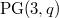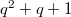﻿

### Cones in$\mathrm{PG}(3, q)$

#### Abstract

In this paper, sets of points of$\mathrm{PG}(3,q)$ of size$q^2+q+1$ and intersecting every plane in$1$,$m$ or$n$ points are studied.

DOI Code: 10.1285/i15900932v40n1p81

Keywords: projective space; ovals; quadratic cones; intersection number

Full Text: PDF